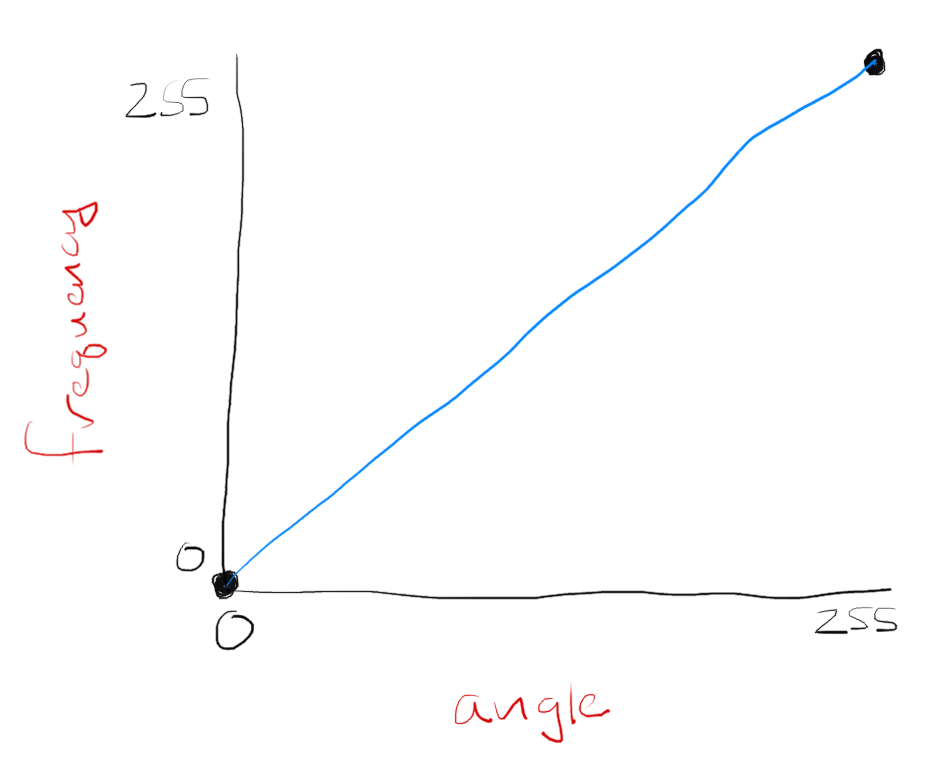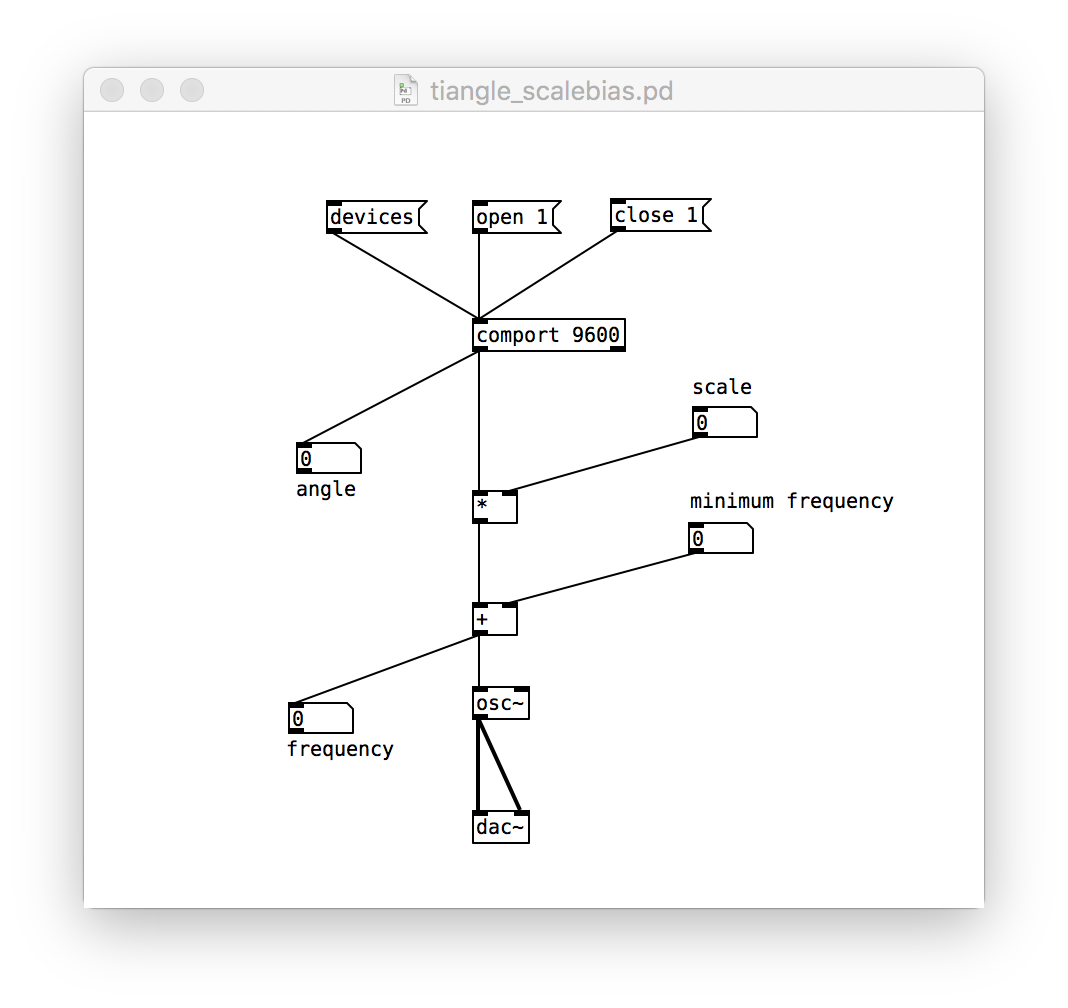# teaching machines

## The Tiangle, Part III

This post is part of a series of notes and exercises for a summer camp on making musical instruments with Arduino and Pure Data.

We now have physical control over the sound waves that are being produced. The only hitch is that a lot of the frequencies in [0, 255] are outside the range that we can hear, and 255 different frequencies is a pretty narrow range. Let’s modify the patch to emit a wider and more audible range of frequencies.

### Fine Tuning the Frequencies

Right now, we’ve got this relationship between the potentiometer’s angle and the emitted frequency:What mathematical function describes this relationship between angle and frequency?
It’s a straight diagonal line, which we usually describe as
$$y = x$$
But in computing, we can and should use more meaningful names:
$$\textrm{frequency} = \textrm{angle}$$

We must tweak this function in two ways to achieve a better range. First, we want to push the frequencies up the y-axis away from the inaudible 0.

How do you push a function up the y-axis?
You add an offset. We often call it b or the y-intercept. Our function changes to this:
$$y = x + b$$
Or, if we’re using better names:
$$\textrm{frequency} = \textrm{angle} + \textrm{minimum frequency}$$

Second, we want to expand the range beyond a mere 255 values.

How do we widen the range of the function?
You multiply by a scale factor. We often call it m or the slope of the line. Our function changes to this:
$$y = mx + b$$
Or, if we’re using better names:
$$\textrm{frequency} = \textrm{scale} \cdot \textrm{angle} + \textrm{minimum frequency}$$

We model our expanded function in Pure Data in this way:See how the 0-255 reading leaves comport and goes through a gauntlet of arithmetic? First it gets scaled and then it gets offset.

Adjust your patch to reflect these changes. Then leave Edit Mode and test things out. Click and drag on the scale and minimum offset widgets in the patch to alter the function that maps the potentiometer’s angle to the emitted frequency.

### Challenges

After you get your improved ranges working, answer the following questions on a piece of scratch paper.

1. How can you adjust the parameters so that turning the potentiometer has no effect on the emitted frequency?
2. What minimum frequency and scale factor do you need to produce frequencies in the range [261, 1047]? (This range roughly goes from middle C to C in the sixth octave.)
3. How can you adjust the parameters so that the potentiometer works the opposite direction? That is, when you crank it clockwise, it behaves like it was being turned counter-clockwise?